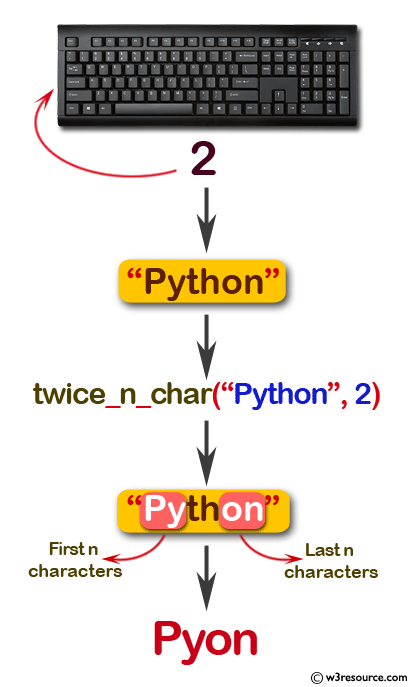﻿ Swift String Programming Exercise: Create a new string made of the first and last n chars from a given string - w3resource# Swift String Exercises: Create a new string made of the first and last n chars from a given string

## Swift String Programming: Exercise-14 with Solution

Write a Swift program to create a new string made of the first and last n chars from a given string. The string length will be at least n.

Pictorial Presentation:Sample Solution:

Swift Code:

``````func twice_n_char(_ input: String, _ n: Int) -> String {
let part1 = String(input.characters.prefix(n))
let part2 = String(input.characters.suffix(n))

return part1 + part2
}
print(twice_n_char("Python", 2))
print(twice_n_char("Swift Examples", 3))
print(twice_n_char("Swift Examples", 1))
```
```

Sample Output:

```
Pyon
Swiles
Ss
```

Swift Programming Code Editor:

Improve this sample solution and post your code through Disqus

What is the difficulty level of this exercise?

﻿